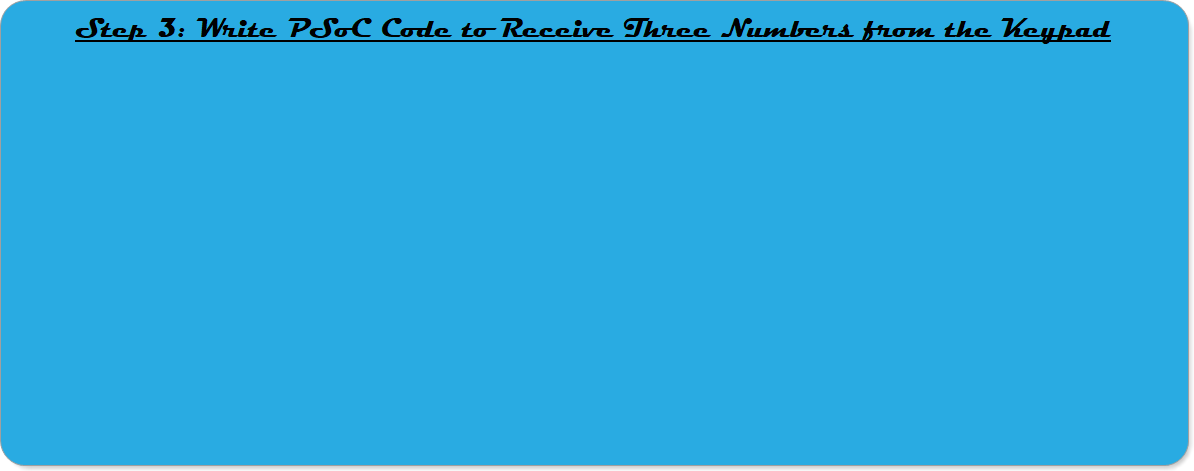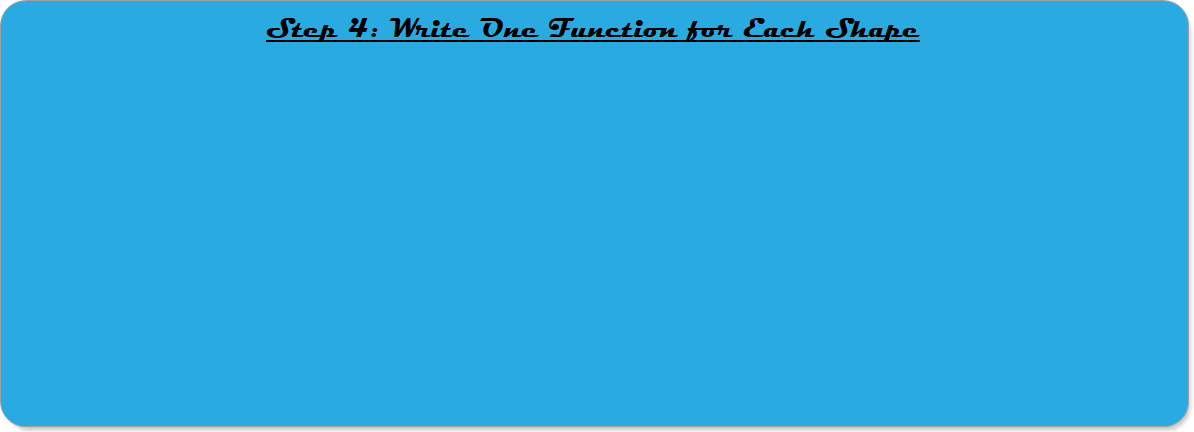Robotics 2
Final Challenge: SCARA WriterYou have now made it through the entire Robotics 2 course, and you are about to test your complete set of knowledge by making a working robotic system.  You've already learned everything you need to know to do this; you just need to put it all together.  In this class, you get to pick one of three options for your Final Challenge.  This option is called the 'SCARA Writer', and it will mostly test your knowledge of kinematics.  You should start by building a SCARA manipulator with the marker as the end-effector.  Here is what the final SCARA Writer should do:

1. First, you run the PSoC code.  PSoC will wait quietly until it receives three values from the first row of the keypad.
2. The first value is a shape indicator.  If the value is 1, that means 'square'.  If the value is 2, that means 'triangle'.  If the value is 3, that means 'circle'.
3. The second value is a size indicator in units of cm.  So, if the shape is square and the size is 2, this indicates a square with side-length 2.  If the shape is circle and the size indicator is 3, this indicates a circle with radius of 3cm.
4. The third value is a speed indicator in units of cm/s.
5. Upon receiving the three values, PSoC will position the marker tip at the 'start' location (which you should have hard-coded).  Then, PSoC will use the Jacobian, path-planning, and trajectory generation to move the marker along the shape path at the specified velocity until the shape is complete.

In order to accomplish this, I recommend that you do the following five steps in order.  As you go, TEST EACH STEP alone, then test each step working together with all of the previous steps.  Don't move on to the next step until you have verified that the current step is working right.

There are five steps, and five in-class days to work on this.  Try as best as you can to keep up with the schedule, and you should be ready to go by the time Finals Week arrives.

The location where the manipulator STARTS to draw the shape can be a constant, and you get to pick where that location is.  Or, you can use a different start location for each shape, or you can pick a different start location depending on the size of the object.  In any case, you want to pick the start location to try and maximize the amount of workspace that you have available for drawing the shape.

If you want to specify the start location in X,Y coordinates, you will have to have some inverse kinematics equations to figure out the servo angles that will put the end-effector in that location.  But, you aren't required to do that - you can just set the angles of the servos to a hard-coded starting location, if you want.

There was a 'bonus' lab where we learned how to use a keypad.  If you didn't do that bonus lab but you selected this Final Challenge, you will have to go learn how to use the keypad in the last video here.

Since you don't want your code to move on until you have received three numbers from the keypad, you might want to write three loops, each of which will keep executing until a number is received, like this:

First_Number=0;
while(First_Number==0)
Second_Number=0;
while(Second_Number==0)
Third_Number=0;
while(Third_Number==0)

We learned in the Jacobian lab and the Path-planning lab that we can follow any kind of path as long as we can write a parametric equation for the path, then use time as the parameter.  Then, we can take the time derivative to find the X and Y velocities.  Then, we can specify the overall velocity of the end-effector with V=sqrt(Xdot^2+Ydot^2).

To make a whole shape, we just have to put multiple paths together.  For example, a square is four straight-line paths.  A triangle is three straight-line paths, and a circle is just one circle path (with a sine and a cosine in the X and Y equations).

Once you have the paths and the velocities figured out, the last thing you need is to make each path stop at the right time.  I think the easiest way to do that is to first figure out how much time it will take to make the path the right length, then use a while loop that is timed.

You could put all of this into your main loop, but I think it's easier to create one function for a square, one function for a triangle, and one function for a circle.  The function would take in two values: one for the size of the shape and one for the end-effector velocity.  The function would then run the loops with the Jacobian matrix in them to make the right paths.
This is the step where you test everything together and fix whatever remaining problems you have.  You need to call the right function at the right time so that the correct shape is produced, and you should at least check these things:

Measure the shapes that are drawn to make sure they are at least close to the right size.  You should shoot for being accurate to within 1 cm.  For example, if the user indicates they want a square that is 3cm on a side, you should produce a square that is between 2 and 4 cm on a side.

Use a stopwatch to find out if the end-effector is moving at the right velocity.  For example, if the user wants a square that is 3cm on a side at a velocity of 1cm/s, use a stopwatch to see if it really takes 3 seconds to make each side, and about 12 seconds to make the whole square.  Shoot for no more than 20% error in the timing.  For example, if the whole square should take 12 seconds, you should time it as 12 seconds +- 2.4 seconds.
This part should be pretty easy, because we've done this step a number of times before.  Your manipulator should look like the one we built in the first video here.  You will want to make sure that the marker lines up with the link it is attached to, with no offset, just like we did in the Final Challenge in the first semester.  Also, you will have more success if you set up the range of angles for the second servo so that it can rotate all the way back on itself.  In other words, the manipulator should be totally straight when all angles are set to 0, and both servos should have a range of 0 to 180 degrees, not -90 to 90.